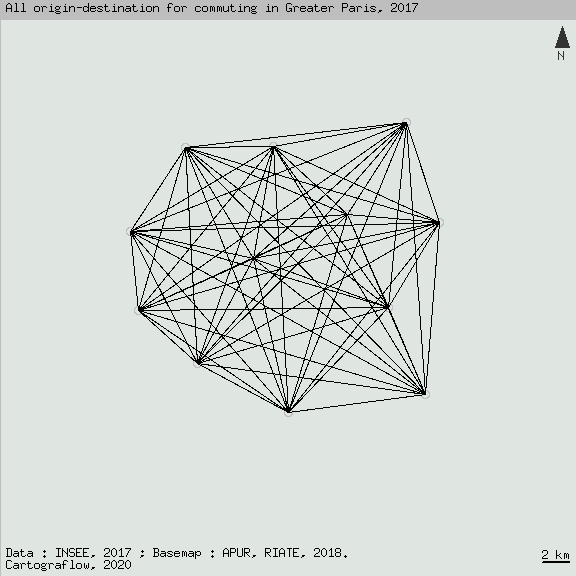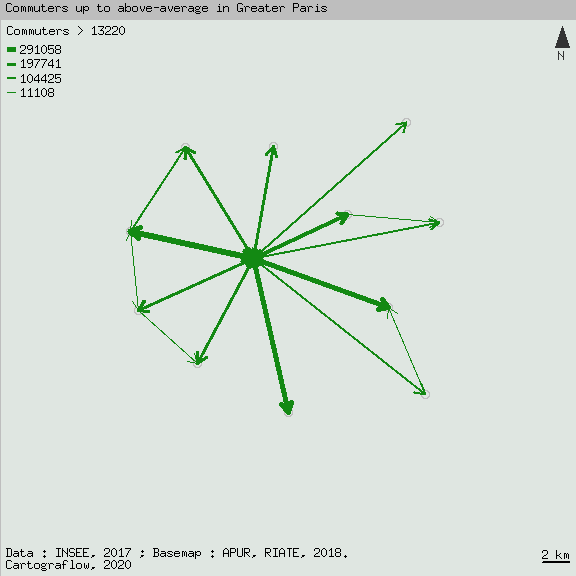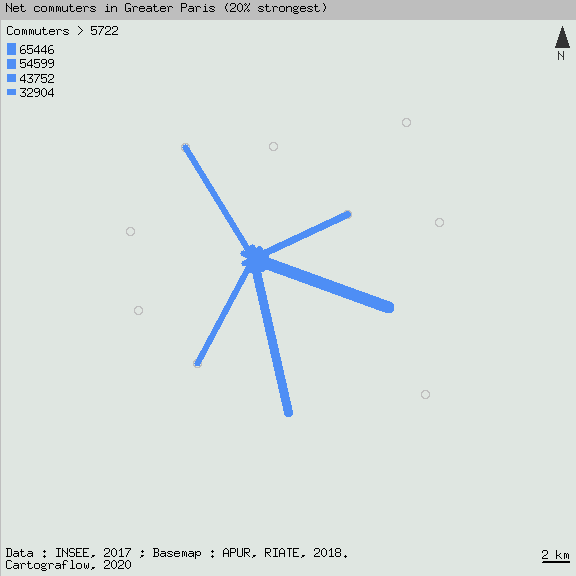# Cartograflow

### Filtering Origin-Destination Matrix for Thematic Flow Mapping

#### 2020-07-19

Cartograflow is designed to filter origin-destination (OD) flow matrix for thematic mapping purposes.

## Description of functions

1. Preparing flow data sets:

1.1 General functions
You can use long “L” or matrix “M” [n*n] flow dataset formats.

flowtabmat() is to transform “L” to “M” formats, also to build an empty square matrix from spatial codes.

flowcarre() is to square a matrix.

flowjointure() is to performs a spatial join between a flow dataset and a spatial features layer or an external matrix.

flowstructmat() fixes an unpreviously codes shift in the flow dataset “M” format. If necessary this function is to be used with flowjointure and flowtabmat.

1.2. Flow computation:

flowtype() is to compute several types of flow from an asymmetric matrix:
x= flux for remaining initial flow (Fij)
x= transpose for reverse flow value (Fji)
x= bivolum for bilateral volum, as gross flow (FSij)
x= bibal for bilateral balance, as net flow (FBij)
x= biasym for asymetry of bilateral flow (FAij)
x= bimin for minimum of bilateral flow (minFij)
x= bimax for maximum of bilateral flow (maxFij)
x= birange for bilateral flow range (rangeFij)
x= bidisym for bilateral disymetry as (FDij)

flowplaces() is to compute several types of flow places oriented from an asymmetric:
ie. as a dataframe that describes the flows from Origin / destination point of view
x= ini for the number of incoming links (as in-degree)
x= outi for the number of outcoming links (as out-degree)
x= degi for the total number of links (as in and out degrees)
x= intra for total intra zonal interaction (if main diagonal is not empty
x= Dj for the total flows received by (j) place
x= voli for the total volume of flow per place
x= bali for the net balance of flow per place
x= asyi for the asymetry of flow per place
x= allflowplaces for computing all the above indicators

1.3. Flow reduction:

flowlowup() is to extracts the upper or the lower triangular part of a matrix - preferably for symmetrical matrixes.

x= up for the part above the main diagonal
x= low for the part below the main diagonal

flowreduct() is to reduce the flow dataset regarding another matrix, e.g. distances travelled.

metric is the metric of the distance matrix :
- metric= continuous (e.g. for kilometers)
- metric= ordinal (e.g. for k contiguity)

If the metric is continuous (e.g for filtering flows by kilometric distances travelled), use:

d.criteria is for selecting the minimum or the maximum distance criteria
- d.criteria= dmin for keeping only flows up to a dmin criterion in km
- d.criteria= dmax for selecting values less than a dmax criterion in km

d is the value of the selected dmin or dmax criteria.

Notice that these arguments can be used as a filter criterion in flowmap().

See Cartograflow_distance and Cartograflow_ordinal_distance Vignettes for examples.
URL: https://github.com/fbahoken/cartogRaflow/tree/master/vignettes

2. Flows filtering:

2.1. Filtering from flow concentration analysis

Flow concentration analysis:

flowgini() performs a Gini’s concentration analysis of the flow features, by computing Gini coefficient and plotting interactive Lorenz curve.

To be use before flowanalysis()

See Cartograflow_concentration Vignette for example.
URL: https://github.com/fbahoken/cartogRaflow/tree/master/vignettes

Flow filtering according to a concentration criterion:

flowanalysis() computes filters criterions based on:

• argument critflow is to filter the flows according to their significativity (% of total of flow information) ;
• argument critlink is to filter the flows according to their density (% of total features)

These arguments can be used as filter criterion in flowmap().

See Cartograflow_concentration Vignette for example.
URL: https://github.com/fbahoken/cartogRaflow/tree/master/vignettes

2.2. Spatial / territorial filtering of flows

Flow filtering based on a continuous distance criterion

flowdist() computes a continous distance matrix from spatial features (area or points). The result is a matrix of the distances travelled between ODs, with flows filtered or not.

See Cartograflow_distance Vignette for example.
URL: https://github.com/fbahoken/cartogRaflow/tree/master/vignettes

Flow filtering based on an ordinal distance / neighbourhood criterion:

flowcontig() compute an ordinal distance matrix from spatial features (area). The result is a matrix of adjacency or k-contiguity of the ODs.

• background is the areal spatial features ;
• code is the spatial features codes ;
• k is to enter the number (k:1,2,…,k) of the contiguity matrix to be constructed : if (k=1), ODs places are adjacent, then the flow have to cross only 1 boundary, else (k=k) ODs places are distant from n borders ;
• algo is the algorithm to use for ordinal distance calculation (also Default is “automatic” for “Dijkstra’s”) ;

Notice that the function automatically returns the maximum (k) number of the spatial layer.

See Cartograflow_distance_ordinal Vignette for example.

3. Flow mapping

flowmap() is to plot flows as segments or arrows, by acting on the following arguments:

• filter is to filter or not flow’s information or features
• threshold is used to set the filtering level of the flows when filter=“True”
• taille is the value of the width of the flow feature
• a.head is the arrow head parameter (in, out, in and out)
• a.length is the length of the edges of the arrow head (in inches)
• a.angle is the angle from the shaft of the arrow to the edge of the arrow head
• a.col is the arrow’s color
• plota is to add spatial features as map background to the flows’s plot
• add is to allow to overlay flow features on external spatial features background

## Examples of applications

Useful packages Best external R package to use: {dplyr} {sf} {igraph} {rlang} {cartography}

## 1. Load datasets

Flow dataset

# Load Statistical information
tabflow<-read.csv2("./data/MOBPRO_ETP.csv", header=TRUE, sep=";",stringsAsFactors=FALSE,
encoding="UTF-8", dec=".",check.names=FALSE)
## 'data.frame':    121 obs. of  4 variables:
##  $i : chr "T1" "T1" "T1" "T1" ... ##$ j    : chr  "T1" "T10" "T11" "T12" ...
##  $Fij : num 291058 8297 3889 17064 12163 ... ##$ count: num  351 43 13 77 52 55 134 63 53 14 ...

Select variable and change matrix format

# Selecting useful variables for changing format
tabflow<-tabflow %>% select(i,j,Fij)

# From list (L) to matrix (M) format
matflow <-flowtabmat(tabflow,matlist="M")
head(matflow[1:4,1:4])
##         T1   T10   T11   T12
## T1  291058  8297  3889 17064
## T10  73743 19501 11707  4931
## T11  22408  9359 12108  6084
## T12  68625  1906  7269 46515
dim(matflow)
##  12 12

Geographical dataset

## 2. Flow types computing

Compute bilateral flows types : eg. volum, balance, bilateral maximum and all types

# Bilateral volum (gross) FSij:
tabflow_vol<-flowtype(tabflow, format="L", origin="i", destination="j", fij="Fij",  x= "bivolum" )
# Matrix format (M= : matflow_vol<-flowtype(matflow, format="M", "bivolum")

# Bilateral balance (net ) FBij:
tabflow_net<-flowtype(tabflow, format="L", origin="i", destination="j", fij="Fij", x="bibal")

# Bilateral maximum (maxFij):
tabflow_max<-flowtype(tabflow, format="L", origin="i", destination="j", fij="Fij", x="bimax")

# Compute all types of bilateral flows, in one 11 columns
tabflow_all<-flowtype(tabflow,format="L", origin="i", destination="j", fij="Fij", x="alltypes")
head(tabflow_all) 
##    i   j    Fij    Fji   FSij  FBij      FAij minFij maxFij rangeFij      FDij
## 1 T1  T1 291058 291058 582116     0 0.0000000 291058 291058        0 0.0000000
## 2 T1 T10   8297  73743  82040 65446 0.7977328   8297  73743    65446 0.7977328
## 3 T1 T11   3889  22408  26297 18519 0.7042248   3889  22408    18519 0.7042248
## 4 T1 T12  17064  68625  85689 51561 0.6017225  17064  68625    51561 0.6017225
## 5 T1  T2  12163  47427  59590 35264 0.5917771  12163  47427    35264 0.5917771
## 6 T1  T3  32682  45772  78454 13090 0.1668494  32682  45772    13090 0.1668494

## 3. Direct flow mapping

3.1. Plot all origin-destination without any filtering criterion The result will reveal a graphic complexity (“spaghetti-effect”")

Plot links

library(sf)
map<-st_read("./data/MGP_TER.shp")
## Reading layer MGP_TER' from data source /tmp/RtmpmeYsUo/Rbuild751f7853470a/cartograflow/vignettes/data/MGP_TER.shp' using driver ESRI Shapefile'
## Simple feature collection with 12 features and 14 fields
## geometry type:  POLYGON
## dimension:      XY
## bbox:           xmin: 637297.4 ymin: 6838629 xmax: 671752.1 ymax: 6879246
## projected CRS:  Lambert_Conformal_Conic
# Add and overlay spatial background
par(bg = "NA")

# Graphic parameters
par(mar=c(0,0,1,0))
extent <- c(2800000, 1340000, 6400000, 4800000)
resolution<-150

plot(st_geometry(map), col = NA, border=NA, bg="#dfe6e1")
plot(st_geometry(map), col = "light grey", add=TRUE)

# Flowmapping of all links

flowmap(tab=tabflow,
fij="Fij",
origin.f = "i",
destination.f = "j",
bkg = map,
code="EPT_NUM",
nodes.X="X",
nodes.Y = "Y",
filter=FALSE,
add=TRUE
)

library(cartography)

# Map cosmetics
layoutLayer(title = "All origin-destination for commuting in Greater Paris, 2017",
coltitle ="black",
author = "Cartograflow, 2020",
sources = "Data : INSEE, 2017 ; Basemap : APUR, RIATE, 2018.",
scale = 2,
tabtitle = FALSE,
frame = TRUE,
col = "grey"
)
# North arrow
north("topright")3.2. Plot the above-average flows

library(sf)
map<-st_read("./data/MGP_TER.shp")
## Reading layer MGP_TER' from data source /tmp/RtmpmeYsUo/Rbuild751f7853470a/cartograflow/vignettes/data/MGP_TER.shp' using driver ESRI Shapefile'
## Simple feature collection with 12 features and 14 fields
## geometry type:  POLYGON
## dimension:      XY
## bbox:           xmin: 637297.4 ymin: 6838629 xmax: 671752.1 ymax: 6879246
## projected CRS:  Lambert_Conformal_Conic
# Add and overlay spatial background
par(bg = "NA")

# Graphic parameters
par(mar=c(0,0,1,0))
extent <- c(2800000, 1340000, 6400000, 4800000)
resolution<-150

plot(st_geometry(map), col = NA, border=NA, bg="#dfe6e1")
plot(st_geometry(map), col = "light grey", add=TRUE)

# Flow mapping above-average flows
flowmap(tab=tabflow,
fij="Fij",
origin.f = "i",
destination.f = "j",
bkg = map,
code="EPT_NUM",
nodes.X="X",
nodes.Y = "Y",
filter=TRUE,
threshold =(mean(tabflow$Fij)), #mean value is the level of threshold taille=20, a.head = 1, a.length = 0.11, a.angle = 30, a.col="#138913", add=TRUE) # Map Legend legendPropLines(pos="topleft", title.txt="Commuters > 13220 ", title.cex=0.8, cex=0.5, values.cex= 0.7, var=c(mean(tabflow$Fij),max(tabflow$Fij)), lwd=5, frame = FALSE, col="#138913", values.rnd = 0 ) #Map cosmetic layoutLayer(title = "Commuters up to above-average in Greater Paris", coltitle ="black", author = "Cartograflow, 2020", sources = "Data : INSEE, 2017 ; Basemap : APUR, RIATE, 2018.", scale = 2, tabtitle = FALSE, frame = TRUE, col = "grey" ) # North arrow north("topright")3.3. Plot the net flows of bilateral flows #library(sf) map<-st_read("./data/MGP_TER.shp") ## Reading layer MGP_TER' from data source /tmp/RtmpmeYsUo/Rbuild751f7853470a/cartograflow/vignettes/data/MGP_TER.shp' using driver ESRI Shapefile' ## Simple feature collection with 12 features and 14 fields ## geometry type: POLYGON ## dimension: XY ## bbox: xmin: 637297.4 ymin: 6838629 xmax: 671752.1 ymax: 6879246 ## projected CRS: Lambert_Conformal_Conic # Net matrix reduction tabflow_net <- tabflow_net %>% filter(.data$FBij>=0)

# Net matrix thresholding
Q80<-quantile(tabflow_net$FBij,0.95) # Add and overlay spatial background par(bg = "NA") # Graphic parameters par(mar=c(0,0,1,0)) extent <- c(2800000, 1340000, 6400000, 4800000) resolution<-150 plot(st_geometry(map), col = NA, border=NA, bg="#dfe6e1") plot(st_geometry(map), col = "light grey", add=TRUE) # Flow mapping above-average flows flowmap(tab=tabflow_net, fij="FBij", origin.f = "i", destination.f = "j", bkg = map, code="EPT_NUM", nodes.X="X", nodes.Y = "Y", filter=TRUE, threshold = Q80, taille=12, a.head = 1, a.length = 0.11, a.angle = 30, a.col="#4e8ef5", add=TRUE) # Map Legend legendPropLines(pos="topleft", title.txt="Commuters > 5722 ", title.cex=0.8, cex=0.5, values.cex= 0.7, var=c(Q80,max(tabflow_net$FBij)),
lwd=12,
frame = FALSE,
col="#4e8ef5",
values.rnd = 0
)

#Map cosmetic

layoutLayer(title = "Net commuters in Greater Paris (20% strongest)",
coltitle ="black",
author = "Cartograflow, 2020",
sources = "Data : INSEE, 2017 ; Basemap : APUR, RIATE, 2018.",
scale = 2,
tabtitle = FALSE,
frame = TRUE,
col = "grey"
)

# North arrow
north("topright")## Sample datasets

Statistical dataset : - INSEE - Base flux de mobilité (2015) - URL : https://www.insee.fr/fr/statistiques/fichier/3566008/rp2015_mobpro_txt.zip

Geographical dataset :

• municipalities : IGN, GEOFLA 2015 v2.1
• Greater Paris : APUR, UMS 2414 RIATE, 2018.

## See also

https://github.com/fbahoken/cartogRaflow/tree/master/vignettes

– cartograflow_general.html
– cartograflow_concentration.html
– cartograflow_distance.html
– cartograflow_ordinal_distance.hmtl

## Reference

– Bahoken Francoise (2016), Programmes pour R/Rtudio annexés, in : Contribution à la cartographie d’une matrix de flux, Thèse de doctorat, Université Paris 7, pp. 325-346. URL : https://halshs.archives-ouvertes.fr/tel-01273776, pp. 480-520.